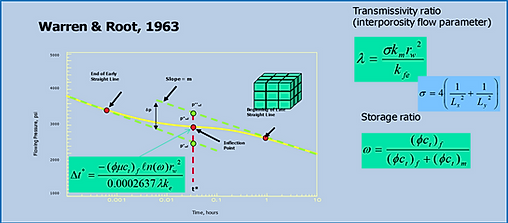top of page

## Matrix-fracture pressure stabilization time

###### ​

This calculator helps to illustrate the importance of fracture intensity (spacing) on matrix-fracture pressure stabilization time, i.e. how quick is the fracture pressure decline at a well impacted by matrix-to-fracture flow.

Input:

• Oil saturation, the fraction of pore volume occupied by oil

• Water saturation, the fraction of pore volume occupied by water

• Matrix and fracture porosity (pore volume % of bulk volume)

• Effective permeability of fracture network and/or matrix

• Matrix and fracture Pore Volume Compressibility (change in pore volume with pore pressure)

• Fracture spacing in x and y (assume idealized, uniform fracture spacing in 2 dimensions)

• Effective total viscosity of fluids in the system

• Oil fluid compressibility (change in oil volume with pore pressure)

• Water compressibility (change in water volume with pore pressure)

• Gas compressibility (change in gas volume with pore pressure)

Note:

rw and kfe have no impact on inflection pointsOutput:

• Calculated total compressibility (pore volume plus total fluid compressibility)

• Calculated matrix shape factor (a function of fracture spacing as per equation)

• Calculated transmissivity ratio (matrix-to-fracture flow capacity relative to fracture only flow capacity as defined in equation)

• Storage ratio (fracture storage  capacity relative to total system storage capacity as defined in equation)

• Inflection time (mid pressure decline inflection  point  between early (fracture only)  and late time (fracture + matrix)  in well pressure decline as defined by equation)

• End of Early St. Line (end of early time well pressure decline  when matrix first impacts well pressure as given by equation )

• Start of 2nd St. Line (start of late time well pressure decline when both matrix and fracture simultaneously impact pressure decline as given by equation)

Symbols in calculator refer to figures above

Author: James R. Gilman

This calculator is not designed to work on mobile devices

JAVASCRIPT MUST BE ENABLED IN YOUR BROWSER FOR THIS CALCULATOR TO WORK PROPERLY

bottom of page